# Fitting Linear Models¶

We work through a case study in this notebook. This notebook accompanies the lecture slides for lecture 23 of DS 100 Fall 2017.

The task is to build a model for predicting the weight of a donkey from more easily obtained measurements.

We are following the work of Milner and Rougier in Significance 2014.

In :
import os
from IPython.display import display, Latex, Markdown

In :
import pandas as pd
import numpy as np
import matplotlib.pyplot as plt
import seaborn as sns

%matplotlib inline

from sklearn import linear_model


We read the data and examine the first few rows and confirm that we have the correct number of rows and columns.

In :
donkeys = pd.read_csv("donkeys.csv")

Out:
BCS Age Sex Length Girth Height Weight WeightAlt
0 3.0 <2 stallion 78 90 90 77 NaN
1 2.5 <2 stallion 91 97 94 100 NaN
2 1.5 <2 stallion 74 93 95 74 NaN
3 3.0 <2 female 87 109 96 116 NaN
4 2.5 <2 female 79 98 91 91 NaN
5 1.5 <2 female 86 102 98 105 NaN
6 2.5 <2 stallion 83 106 96 108 NaN
7 2.0 <2 stallion 77 95 89 86 NaN
8 3.0 <2 stallion 46 66 71 27 NaN
9 3.0 <2 stallion 92 110 99 141 NaN
In :
donkeys.shape

Out:
(544, 8)

## Data Cleaning¶

Weight was measured twice on a sample of 31 donkeys to check the accuracy of the scale. The second weighing is in WeightAlt.

Let's compare the two values by taking the differences.

In :
two_weighings = np.subtract(donkeys['WeightAlt'], donkeys['Weight'])

In :
sns.distplot(two_weighings.dropna())
#plt.savefig("twoweighings.pdf")

Out:
<matplotlib.axes._subplots.AxesSubplot at 0x12693d0f0>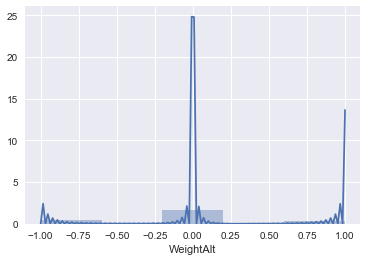The measurements are all within 1 kg of each other.

Next let's look for unusual value that might indicate errors or other problems.

In :
donkeys.describe()

Out:
BCS Length Girth Height Weight WeightAlt
count 544.000000 544.000000 544.000000 544.000000 544.000000 31.000000
mean 2.889706 95.674632 115.946691 101.349265 152.104779 150.258065
std 0.425656 7.348897 7.438570 4.256430 26.506715 22.711183
min 1.000000 46.000000 66.000000 71.000000 27.000000 98.000000
25% 2.500000 92.000000 112.750000 99.000000 139.000000 141.500000
50% 3.000000 97.000000 117.000000 102.000000 155.000000 151.000000
75% 3.000000 101.000000 121.000000 104.000000 170.000000 165.500000
max 4.500000 112.000000 134.000000 116.000000 230.000000 194.000000
In :
donkeys.quantile([0.005, 0.995])

Out:
BCS Length Girth Height Weight WeightAlt
0.005 1.5 71.145 90.000 89.0 71.715 98.75
0.995 4.0 111.000 131.285 112.0 214.000 192.80

There is one emaciated donkey with a BCS (body condition score) of 1 and one overweight donkey with a BCS of 4.5. There is also one very small donkey (28 kg), which on further inspection appears to be a baby donkey. These 3 anamolous cases are dropped from the data frame. The model that we fit will apply to resaonable healthy (BCS between 1.5 and 4) mature donkeys.

We make a copy of the original data set and name it donkeys2.

In :
donkeys2 = donkeys[(donkeys['BCS'] > 1) & (donkeys['BCS'] < 4.5) &
(donkeys['Weight'] > 50)].copy()

donkeys2 = donkeys2.reset_index()

y_donkeys2 = donkeys2['Weight']

In :
donkeys2.head(10)

Out:
index BCS Age Sex Length Girth Height Weight WeightAlt
0 0 3.0 <2 stallion 78 90 90 77 NaN
1 1 2.5 <2 stallion 91 97 94 100 NaN
2 2 1.5 <2 stallion 74 93 95 74 NaN
3 3 3.0 <2 female 87 109 96 116 NaN
4 4 2.5 <2 female 79 98 91 91 NaN
5 5 1.5 <2 female 86 102 98 105 NaN
6 6 2.5 <2 stallion 83 106 96 108 NaN
7 7 2.0 <2 stallion 77 95 89 86 NaN
8 9 3.0 <2 stallion 92 110 99 141 NaN
9 10 3.0 <2 female 86 105 92 100 NaN

## Test-Train split¶

Before we proceed with our data analysis, we divide the data into an 80% - 20% split and set aside 20% for evaluation of the model.

In :
from sklearn.model_selection import train_test_split

#train, test = train_test_split(donkeys2, test_size=0.2, random_state=1141)

In :
indices = np.arange(len(donkeys2))
np.random.shuffle(indices)
n_train = int(np.round((len(donkeys2)*0.8)))
n_test = len(donkeys2) - n_train

In :
indices[:n_train]

Out:
array([470, 485, 373, 326,  55, 413, 197, 195, 249, 151, 262, 468, 338,
228, 525, 455, 424, 371, 290, 110, 407, 421, 426, 127, 454, 344,
132,  90, 506, 452, 315, 274, 396,  53, 175, 360, 300, 369, 236,
518, 263, 178, 191, 531, 345, 465, 447, 476, 185, 205, 162, 169,
204, 339, 500, 271, 478,  99, 351, 536, 335, 186, 218, 147, 159,
521,  68, 287, 317, 358, 377, 453, 130, 406, 540, 242, 314, 168,
283, 292, 190, 423, 100, 383, 303, 148, 534, 273,  67,  83, 170,
357,  33, 451, 203, 462, 208, 416, 507, 361, 321, 207, 502, 418,
306,  40,  76, 493, 359, 192, 199, 387, 137, 458, 456,  94,  62,
116, 515, 114, 318, 238,  32,  80, 295, 294, 425,  22, 252,  29,
117, 189, 355, 427, 448, 144,  51, 106, 432, 411, 311, 135, 112,
342, 240, 497, 152, 259, 272, 121, 469, 380, 291, 417, 390,  12,
26, 239, 222,  73,  84, 224, 409, 459, 126,  19, 480, 430, 235,
438, 233,  10, 141,  31, 265,  89, 181, 378, 270, 284, 299,  37,
187,  17, 389,  61, 256, 487, 414, 400,  79, 182, 352, 535, 202,
395, 499, 408,  75, 301, 391, 475,  45, 143,  35, 372, 278, 103,
524,  13,  86, 157, 375, 119, 460, 146,  38, 123, 293, 477, 164,
269,  97, 150, 494, 420, 184, 140, 331, 340, 399, 155, 496, 145,
422,  23, 122, 160, 212,  15, 213, 289,  82,  69,  43, 433,  21,
88, 188, 180,  54,  78, 322,  96,  72, 231, 166, 393, 163, 347,
319, 288, 362, 429, 107, 245, 109,  49,  70, 443, 124, 247, 504,
39, 498, 125, 444, 257, 229,  60, 261, 483, 328, 297, 120, 237,
63,  77, 277, 356, 370, 334, 385, 374, 440, 379, 267,  44, 158,
484, 200, 486, 392,  98, 353, 527, 220, 513, 402, 445, 341, 471,
431, 519, 193, 533, 307, 258, 313, 457, 111,  42,  85, 214, 134,
298, 153, 154, 108, 133, 467,  50, 279, 381,  14, 118, 217, 466,
302, 503,  30, 113, 172, 464, 139, 250, 129, 449,  71, 450, 333,
397, 248, 439, 441,   7, 437,  52, 479, 539,  25, 537, 511, 343,
5,  87, 522, 436,   4, 230, 488,  41, 264, 246, 481, 142, 323,
320, 309, 398,  91, 489, 234, 211, 495, 350, 538, 149, 435, 266,
48,  65, 308, 330,   3, 281, 394, 365, 364, 179, 505, 520, 285,
81,   1, 523, 194,  66, 403, 368, 138, 514, 253, 482, 176,  74,
131, 412, 254, 384, 226,  16,  18, 241, 490, 532, 442, 310,  58,
386, 201, 227, 167])

## EDA¶

We briefly explore the data in the training set.

We examine the categorical variables with boxplots.

We also examine the relationship between explanatory numberic variables.

In :
train = donkeys2.iloc[indices[ :n_train], ]

In :
sns.boxplot(x = train['BCS'], y = train['Weight'])
#plt.savefig("bcs_box.pdf")

Out:
<matplotlib.axes._subplots.AxesSubplot at 0x126bc67f0>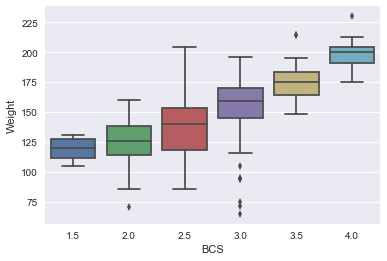In :
sns.boxplot(x = train['Age'], y = train['Weight'],
order = ['<2', '2-5', '5-10', '10-15', '15-20', '>20'])
#plt.savefig("age_box.pdf")

Out:
<matplotlib.axes._subplots.AxesSubplot at 0x126bfc550>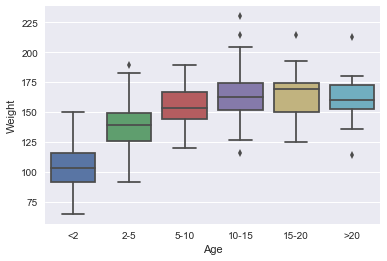In :
sns.boxplot(x = train['Sex'], y = train['Weight'], order = ['female', 'stallion', 'gelding'])
#plt.savefig("sex_box.pdf")

Out:
<matplotlib.axes._subplots.AxesSubplot at 0x126a75748>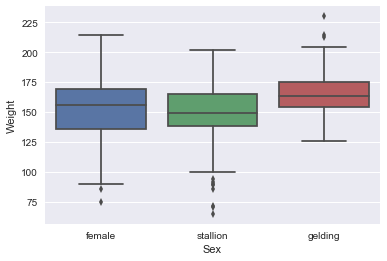In :
sns.regplot('Girth', 'Length', train)
#plt.savefig('girth_length.pdf')

Out:
<matplotlib.axes._subplots.AxesSubplot at 0x126d20f98>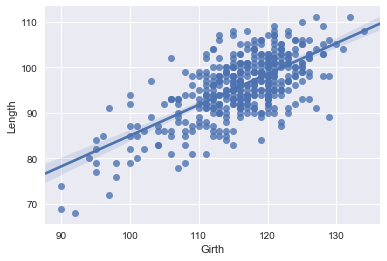Observations:

• Age distribution looks similar for donkeys over 5
• Doesn't appear to be much of a difference in weight for different sexes
• Median weight increase with BCS, but not linearly. (BCS is ordinal, not numeric)

## Starting point for a model¶

We can think of a donkey as a cylinder with appendages. This leads to the mass of the donkey being proportion to a product of the girth and length. This implies a model for log(weight) that is linear in log of girth and length, i.e., $\log(weight) = \alpha + \beta \log(length) + \gamma \log(girth)$.

Milner and Rougier broaden the starting point to consider models of the form:

$$h_\lambda(weight) = X\theta$$

where $h_\lambda(y) = \frac {y^\lambda - 1}{\lambda}$ for $\lambda \neq 0$ and $h_\lambda(y) = log(y)$ for $\lambda = 0$ And where $X$ has 8 possible forms according to whether each of the three variables log(girth), log(height) or log(length) is included.

### Transform the response variables¶

All of these models use the log-transformed values for Length, Height, and Girth. We add their transformed variables to our design matrix.

In :
donkeys2['Length_log'] = np.log(donkeys2['Length'])
donkeys2['Height_log'] = np.log(donkeys2['Height'])
donkeys2['Girth_log'] = np.log(donkeys2['Girth'])


## Choosing the loss function¶

We develop our own loss function to reflect the cost to the donkey's health of an incorrect dose. In particular for anesthetics, we want to err on the side of under dosing because it can be dangerous to over dose and the effects of the drug can be observed, meaning that if the donkey is still in pain then the dose can be increased.

For this reason, we design our own aneshtetic loss function:

In :
def an_loss(x):
wt = (x >= 0) + 3 * (x < 0)
return np.square(x) * wt

In :
xs = np.linspace(-40, 40, 500)
loss = an_loss(xs)
plt.xlabel(r"relative error")
plt.ylabel(r"Loss")
plt.savefig("l1mod_plt.pdf")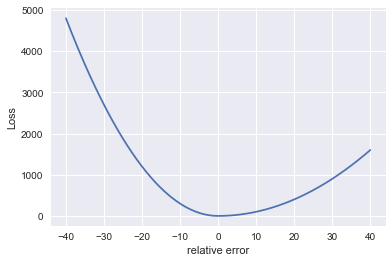Notice that the loss function is expressed in terms of relative error. $$\frac {y - \hat{y}} {\hat{y}}$$ A negative loss indicate an overdose.

## Model Selection¶

We want to fit the best model where we choose the transformation for $y$ and the best subset of the three quantitative variables, girth, length and height. To do this, we fit the 7 possible models (all combinations of the subsets of the three variables) for each $\lambda$. We use our special loss function in the optimization.

### Choosing the Box-Cox transformation¶

We set up a few possible $\lambda$ values, keeping to values that are easily interpretable.

In :
lmbdas = [-1., -0.75, -0.667, -0.5, -0.333, 0, 0.333, 0.5, 0.667, 0.75, 1, 1.5, 2]
len(lmbdas)

Out:
13

We import the stats library which has a Box-Cox tranformation.

In :
from scipy import stats
from scipy.stats import boxcox


We will find it useful to have the inverse of the Box-Cox transformation so that we can compare the predicted values in he original units. We write our own.

In :
def invboxcox(y, ld):
if ld == 0:
return np.exp(y)
else:
y2 = np.where(y > 0, y, 0.0)
return ((ld*y2)+1)**(1/ld)


We import minimize in order to optimize with our special loass function.

In :
# import the optimize library
from scipy.optimize import minimize


In the optimization we want to use the relative error so we revise define a new loss function that incorporates our original loss function.

In :
# Update the loss function to compute relative loss
def new_loss(theta, X, y):
yhat = X @ theta
return np.mean(an_loss((y - yhat) / yhat))


Before we fit all $7 \times 13$ models, we optimize for the two variable model with girth and length and the square-root transformation ($\lambda = 0.5$ ) of weight.

First, we examine therelationship between the transformed weight and log girth.

In :
train = donkeys2.iloc[indices[ :n_train], ]
y_orig = train["Weight"]
y_boxcox = boxcox(y_orig, lmbda = lmbdas)

In :
sns.regplot(x = train['Girth'], y = y_boxcox)
#plt.savefig('girth_length.pdf')

Out:
<matplotlib.axes._subplots.AxesSubplot at 0x1258ec7b8>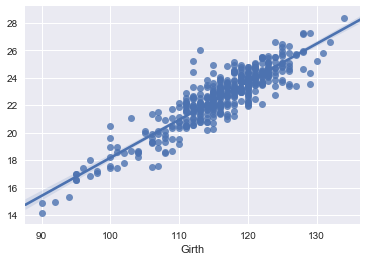In :
X = train[['Girth_log', 'Height_log']]
X_1 = np.hstack([X.values, np.ones((len(X), 1))])

res = minimize(lambda theta: new_loss(theta, X_1, y_boxcox), np.ones(3))

theta_est = res['x']
y_pred = X_1 @ theta_est
y_hat = invboxcox(y_pred, lmbdas)

100* np.sqrt(np.mean(an_loss((y_orig - y_hat)/ y_hat)))
#np.sqrt(np.mean((an_loss((y_orig - y_hat)/ y_hat))))

Out:
9.3448136526278045
In :
100 * np.sqrt(np.mean(an_loss((y_boxcox - y_pred)/ y_pred)))

Out:
5.0482349961037789

Investigate the fit by examing the residuals

In :
resids = y_boxcox - y_pred
plt.scatter(y_pred, resids)

Out:
<matplotlib.collections.PathCollection at 0x12251b128>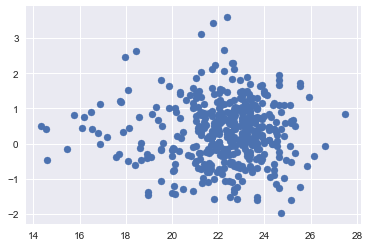Now, we're ready to fit all of the possible models.

To iterate over all possible combinations of explanatory variables and lambdas. We generate the combinations of explanatory variables.

In :
import itertools

models = []
response_vars = ['Girth_log', 'Length_log', 'Height_log']

for L in range(1, len(response_vars)+1):
for subset in itertools.combinations(response_vars, L):
models.append(list(subset))

models

Out:
[['Girth_log'],
['Length_log'],
['Height_log'],
['Girth_log', 'Length_log'],
['Girth_log', 'Height_log'],
['Length_log', 'Height_log'],
['Girth_log', 'Length_log', 'Height_log']]
In :
rmse_mods = []

for a_mod in models:
rmse = []
for lmbda in lmbdas:
y_boxcox = boxcox(y_orig, lmbda = lmbda)
X = train[a_mod]
X_1 = np.hstack([X.values, np.ones((len(X), 1))])
res = minimize(lambda theta: new_loss(theta, X_1, y_boxcox), np.ones(len(a_mod)+1))
theta_est = res['x']
y_pred = X_1 @ theta_est
y_hat= invboxcox(X_1 @ theta_est, lmbda)
#        rmse.append(100*np.sqrt(np.mean(((y_orig - y_hat)/ y_hat)**2)))
rmse.append(100*np.std((y_orig - y_hat)/ y_hat))
rmse_mods.append(rmse)

In :
rmse_mods

Out:
[7.395140637258686,
6.988521226680097,
6.883213591489948,
6.700585917659599,
6.557115443759722,
6.374250754822134,
6.325965463543044,
6.363821583656327,
6.443983676461884,
6.5031026936640925,
6.749914532883333,
16.365937201083206,
16.4013157440236]
In :
for i in range(0, 7):
plt.plot(lmbdas, rmse_mods[i], "-o", label = models[i])

plt.xlabel(r"lambda")
plt.ylabel("Average Loss")
plt.legend(loc = "best")
plt.savefig("lossFunction6.pdf")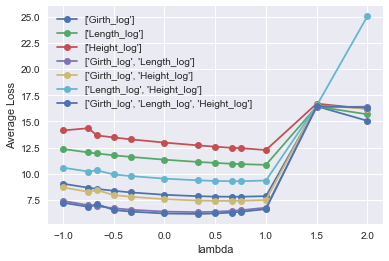The three-variable model has slightly smaller error than the girth-length model. We will stick with the two-variable model as there is little gain from the additional variable.

The best $\lambda$ is $\lambda = 1/3$, but when we compare the error rate for $\lambda = 0, 1/3, 1/2$, we see that they differs by less than 0.03 so we choose the log transformation.

## Transforming Categorical Variables¶

Next we transform the categorical variables into dummy variables so that we can include them in the model.

In :
donkeys2 = pd.get_dummies(donkeys2, columns=["BCS", "Age", "Sex"])

In :
donkeys2.head()

Out:
index Length Girth Height Weight WeightAlt Length_log Height_log Girth_log BCS_1.5 ... BCS_4.0 Age_10-15 Age_15-20 Age_2-5 Age_5-10 Age_<2 Age_>20 Sex_female Sex_gelding Sex_stallion
0 0 78 90 90 77 NaN 4.356709 4.499810 4.499810 0 ... 0 0 0 0 0 1 0 0 0 1
1 1 91 97 94 100 NaN 4.510860 4.543295 4.574711 0 ... 0 0 0 0 0 1 0 0 0 1
2 2 74 93 95 74 NaN 4.304065 4.553877 4.532599 1 ... 0 0 0 0 0 1 0 0 0 1
3 3 87 109 96 116 NaN 4.465908 4.564348 4.691348 0 ... 0 0 0 0 0 1 0 1 0 0
4 4 79 98 91 91 NaN 4.369448 4.510860 4.584967 0 ... 0 0 0 0 0 1 0 1 0 0

5 rows × 24 columns

Drop the variables that we are not using: 'Length" and 'WeightAlt'. Also drop one category from 'Sex', 'BCS' and 'Age' dummies so that the matrix is not over paramterized.

In :
donkeys2 = donkeys2.drop(['index', 'Height',  'Length', 'Girth', 'Weight',
'Height_log', 'WeightAlt',
'BCS_3.0', 'Age_5-10', 'Sex_female'],
axis = 1)

In :
donkeys2.head(10)

Out:
Length_log Girth_log BCS_1.5 BCS_2.0 BCS_2.5 BCS_3.5 BCS_4.0 Age_10-15 Age_15-20 Age_2-5 Age_<2 Age_>20 Sex_gelding Sex_stallion
0 4.356709 4.499810 0 0 0 0 0 0 0 0 1 0 0 1
1 4.510860 4.574711 0 0 1 0 0 0 0 0 1 0 0 1
2 4.304065 4.532599 1 0 0 0 0 0 0 0 1 0 0 1
3 4.465908 4.691348 0 0 0 0 0 0 0 0 1 0 0 0
4 4.369448 4.584967 0 0 1 0 0 0 0 0 1 0 0 0
5 4.454347 4.624973 1 0 0 0 0 0 0 0 1 0 0 0
6 4.418841 4.663439 0 0 1 0 0 0 0 0 1 0 0 1
7 4.343805 4.553877 0 1 0 0 0 0 0 0 1 0 0 1
8 4.521789 4.700480 0 0 0 0 0 0 0 0 1 0 0 1
9 4.454347 4.653960 0 0 0 0 0 0 0 0 1 0 0 0
In :
train = donkeys2.iloc[indices[:n_train], ]
y_train = y_donkeys2[indices[ :n_train]]
print("length of train", len(train))


length of train 433

Out:
470    173
485    159
373    141
326    164
55     133
413    178
197    140
195    155
249    150
151    161
Name: Weight, dtype: int64
In :
X_1 = np.hstack([train.values, np.ones((len(train), 1))])
y_boxcox = boxcox(y_train, 0)

In :
res = minimize(lambda theta: new_loss(theta, X_1, y_boxcox), np.ones(X_1.shape))

In :
theta_est = res['x']
theta_est

Out:
array([  7.45183531e-01,   1.66556953e+00,  -6.61834833e-02,
-4.60638157e-02,  -2.13313745e-02,   3.79782685e-02,
7.92848480e-02,   1.05003285e-03,   9.91633699e-03,
-2.46686048e-02,  -7.94213108e-02,   4.27731276e-03,
8.53325462e-03,   1.91415499e-02,  -6.32111957e+00])
In :
y_pred = X_1 @ theta_est
y_pred[:10]

Out:
array([ 5.15811189,  5.02990018,  4.90585761,  5.03273335,  4.94895807,
5.15650369,  4.87241177,  5.04501052,  5.04867573,  5.01872866])

Let's examine the coefficients for the dummy variables to determine whether we want to keep them in the model or if we want to collapse into fewer categories.

In :
theta_est[[2, 3, 4, 5, 6, 10,9, 7, 8, 11, 12, 13]]

Out:
array([-0.06618348, -0.04606382, -0.02133137,  0.03797827,  0.07928485,
-0.07942131, -0.0246686 ,  0.00105003,  0.00991634,  0.00427731,
0.00853325,  0.01914155])
In :
labels = ['1.5','2.0','2.5','3.5','4.0','<2','2-5', '10-15','15-20','>20', 'ge', 'st']

plt.scatter(x = range(12),
y = theta_est[[2, 3, 4, 5, 6, 10,9, 7, 8, 11, 12, 13]])

plt.xticks(range(12), labels)
plt.axvline(x=4.5)
plt.axvline(x = 9.5)

Out:
<matplotlib.lines.Line2D at 0x123de2128>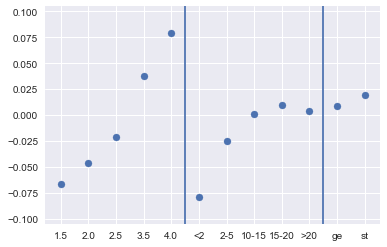The plot of coefficients above indicates that we could collapse the age catgories into 3: under 2, 2 to 5, and over 5. We also see that the coefficients for the sex dummies are close to 0 so we won't lose much if we drop the sex variable from the model. Ideally we would want to compare the size of these coefficients to their standard errors, but we won't do that here.

## Final Model¶

We can drop all of the sex dummies. We can also drop the dummies for age over 10 and then the base age category will be for age over 5.

In :
donkeys2 = donkeys2.drop(['Age_10-15', 'Age_15-20', 'Age_>20',
'Sex_gelding', 'Sex_stallion'],
axis = 1)

In :
train = donkeys2.iloc[indices[:n_train], ]


Fit the new model to the training set.

In :
X_1 = np.hstack([train.values, np.ones((len(train), 1))])
res = minimize(lambda theta: new_loss(theta, X_1, y_boxcox), np.ones(X_1.shape))

In :
theta_est = res['x']
theta_est

Out:
array([ 0.72369783,  1.65854789, -0.06226638, -0.04044221, -0.02232754,
0.03867827,  0.08235653, -0.02728433, -0.08640164, -6.17902583])

## Evaluate the model¶

Finally, we use the fitted model to predict the weights for those donkeys in the test set. First, we get the test set

In :
test_set = donkeys2.iloc[indices[n_train:], ]
y_test = y_donkeys2[indices[n_train:]]
print("length of test", len(test))

X_test_1 = np.hstack([test_set.values, np.ones((len(test_set), 1))])
y_boxcox_test = boxcox(y_test, 0)

length of test 109


Now we estimate the transformed weight from the model fitted to the training data, and we convert the predicted values into original units.

In :
y_test_pred = X_test_1 @ theta_est
y_test_pred_o = invboxcox(y_test_pred, 0)


The following plot shows the typical errors. The red lines correspond to plus/minus 10% of the predicted weight.

In :
plt.scatter(y_test_pred_o, y_test)
plt.plot([75, 225], [75, 225], 'k-', color = 'r')
plt.plot([75, 225], [82.5, 247.5], 'k-', color="red")
plt.plot([75, 225], [67.5, 202.5], 'k-', color="red")
plt.xlabel("Predicted Weight (kg)")
plt.ylabel("Actual Weight (kg)")
plt.savefig("model_assess.pdf")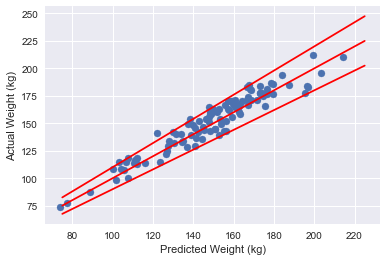Nearly all the predicted weights are within 10% of the actual weights. Below we calculate the percentage that are within 10%.

In :
100* np.sum(np.abs((y_test - y_test_pred_o )/y_test_pred_o) < 0.1) / len(y_test)

Out:
95.370370370370367